May 26, 2016

## Reproducibility

There are 3 basic concepts of reproducibility in research:

1. Reproducible experiments

2. Reproducible analysis

3. Reproducible inference

## Reproducibility

There are 3 basic concepts of reproducibility in research:

1. Reproducible experiments

Another lab can do the same experiment and obtain similar results.

2. Reproducible analysis

Another analyst can redo the analysis with the same data and obtain identical results.

3. Reproducible inference

After reproducing the experiment in another lab, similar scientific inferences will be made.

## Reproducibility

Today:

• How selection bias leads to irreproducibility
• How simulation can help us assess statistical validitiy
• How to set up synthetic data that mimics actual data
• Using synthetic data to assess statistical significance

## Example S1

We have done an experiment in which we measured a phenotypic score on each sample (e.g. biopsy result) and also measured the gene expression for 1000 genes in the samples.

Our objective is to determine which genes are associated with the phenotypic score, and to develop a prediction equation for the phenotype

## Example S2

We pursue 2 analyses:

1. Correlation between gene expression and phenotypic score.

2. Division of the samples into "low" and "high" scores, followed by a t-test to determine if there is a difference in gene expression in the low and high score groups.

## Example S3

There are some genes with correlation as low as -0.6 or as high as 0.6 with the phenotype.

These are good candidates for genes that are important to determining or predicting genotype.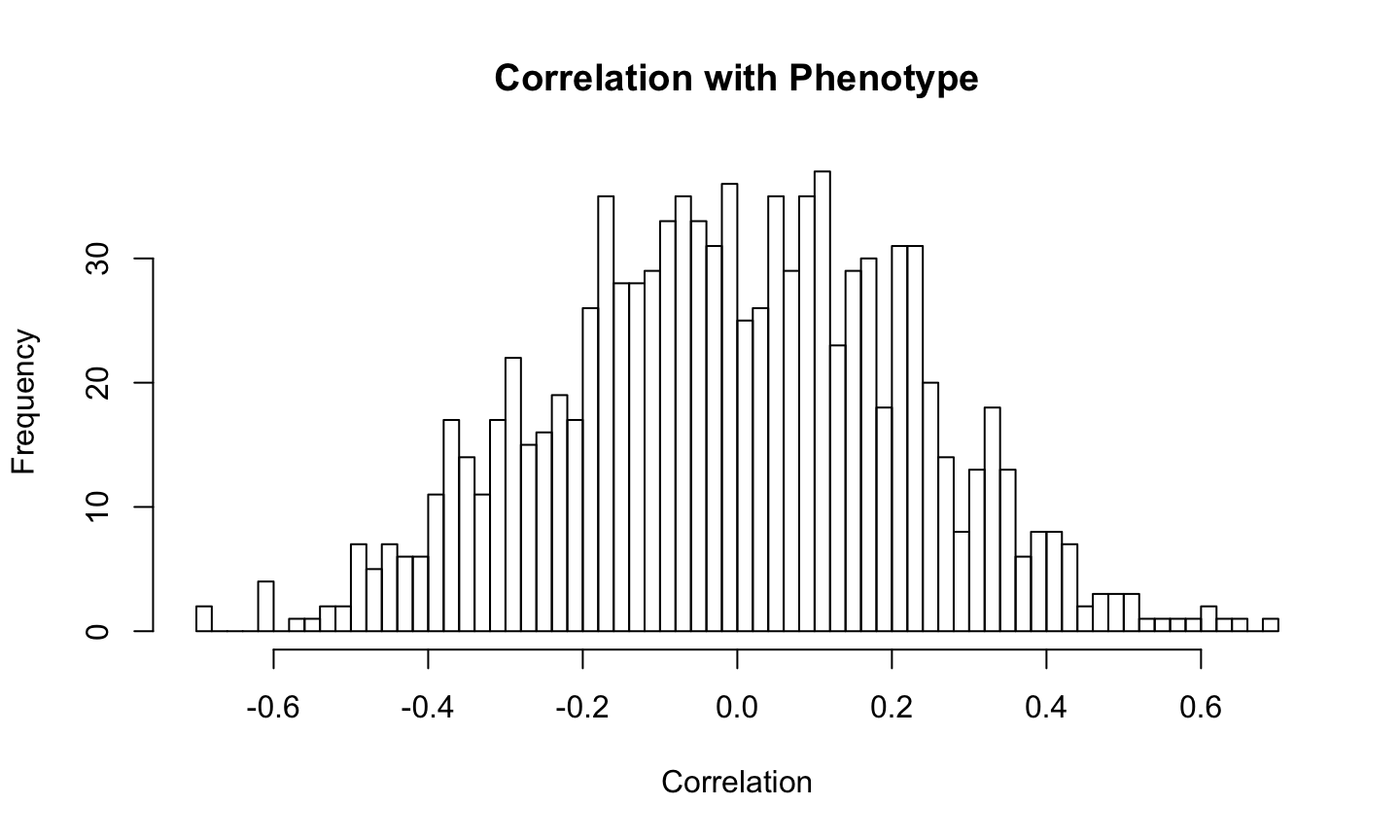## Example S4

Lets select the 10 genes with the highest absolute correlation and see how well they predict phenotypic score.

```anov=anova(regfit)
anov```
```## Analysis of Variance Table
##
## Response: pheno
##           Df  Sum Sq Mean Sq F value    Pr(>F)
## sigGenes  10 25.5240 2.55240  14.074 0.0002485 ***
## Residuals  9  1.6322 0.18136
## ---
## Signif. codes:  0 '***' 0.001 '**' 0.01 '*' 0.05 '.' 0.1 ' ' 1```

Note that \(R^2=\) 0.94 and the p-value is 2.4810^{-4} which are highly significant.

## Example S5

Now lets do an alternative analysis classifying the phenotype and "low" and "high" and using an eBayes t-test to distinguish between them.

Note that this is a 2-sample t-test with 18 d.f. so the values greater than 2.1 (or less than -2.1) are significant at p<.05. There are several of these.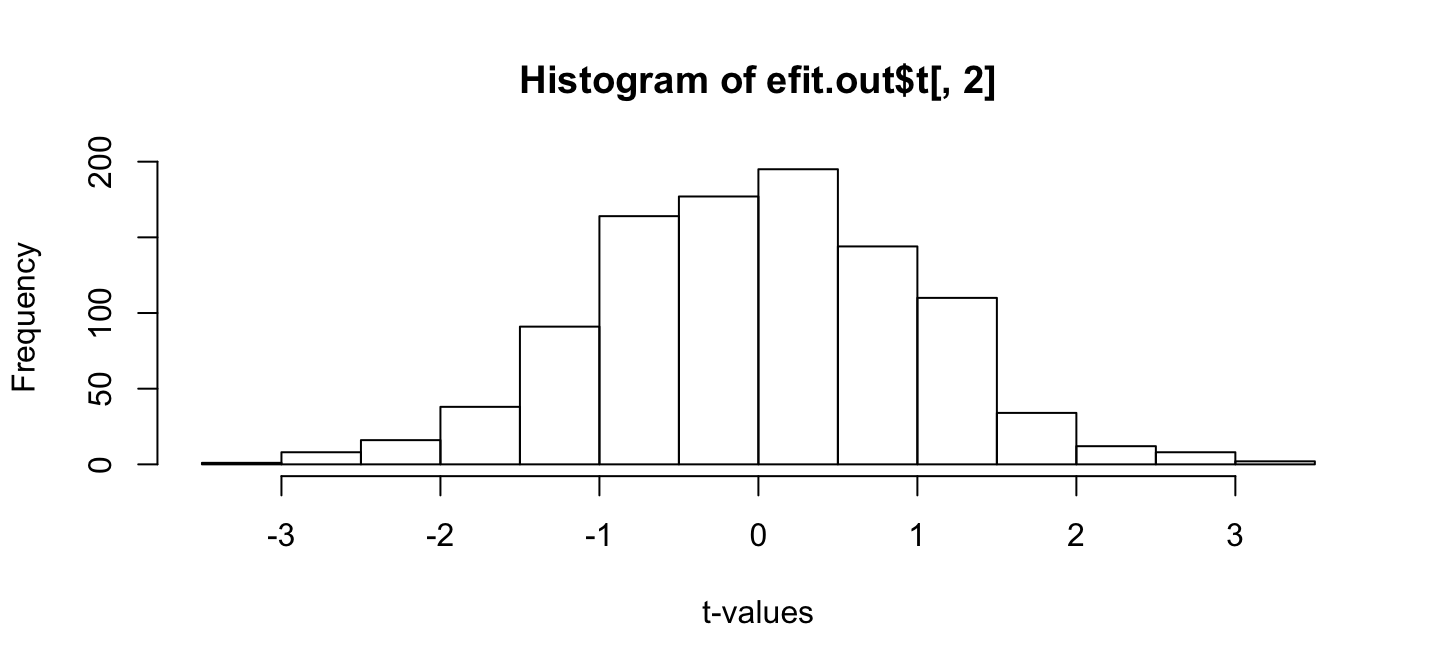## Example S6

We might also wonder if the most statistically significant genes in this analysis match the ones from the correlation analysis.

`plot(cors,efit.out\$t[,2],xlab="Correlation",ylab="t-statistic")`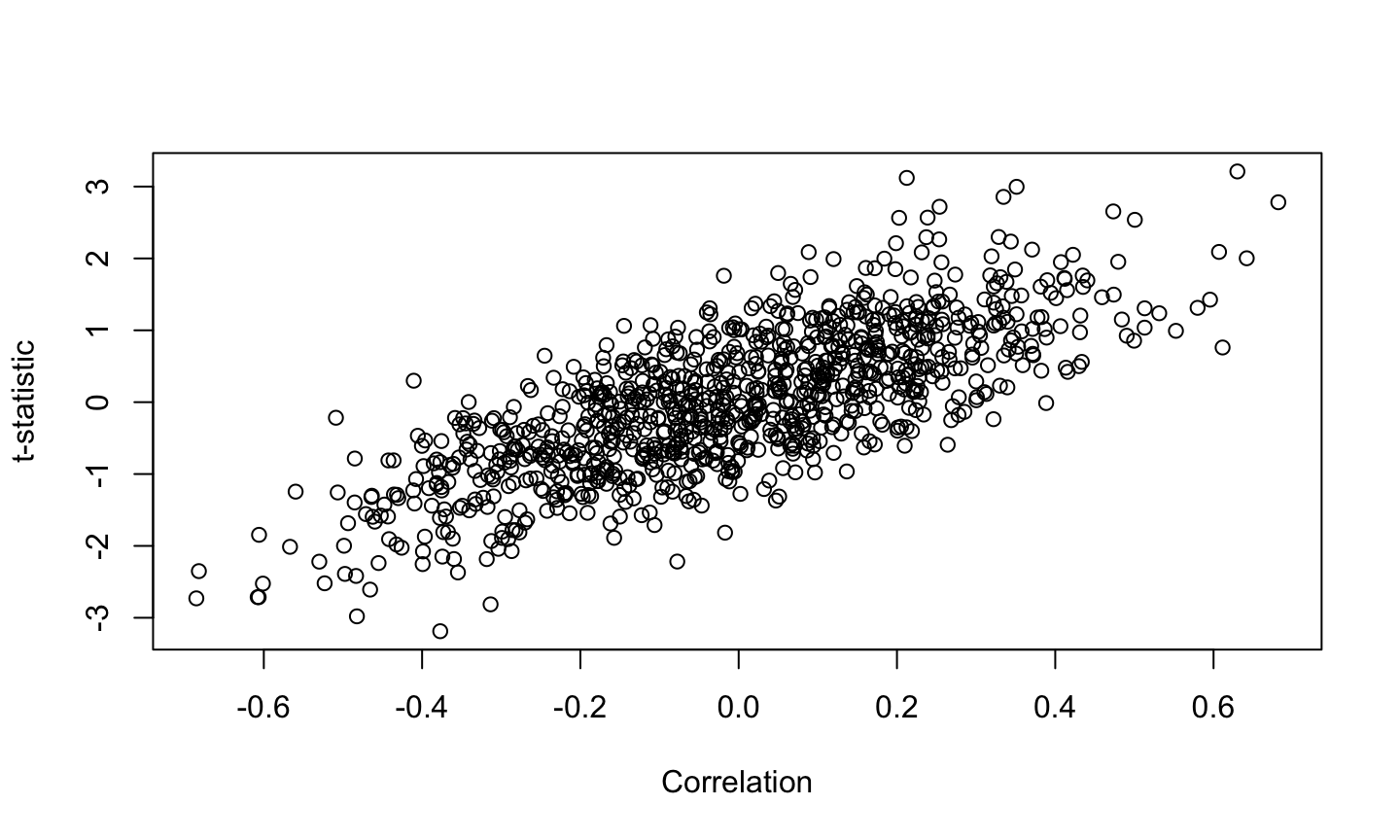We see that there is very high correlation between the correlation statistic and the t-statistic, so that almost the same gene set will be selected.

## Example S7

Naturally, we feel that we have done a great job of this experiment, but our collaborator wants to redo it to verify.

We compare results:

## Example S8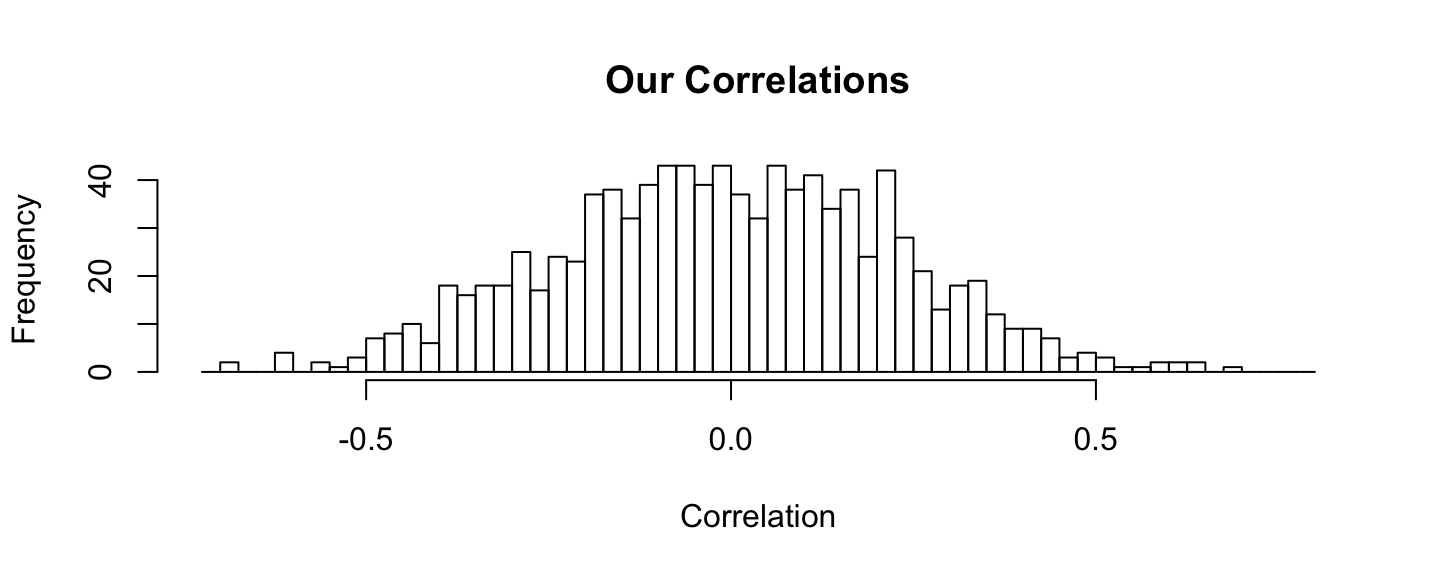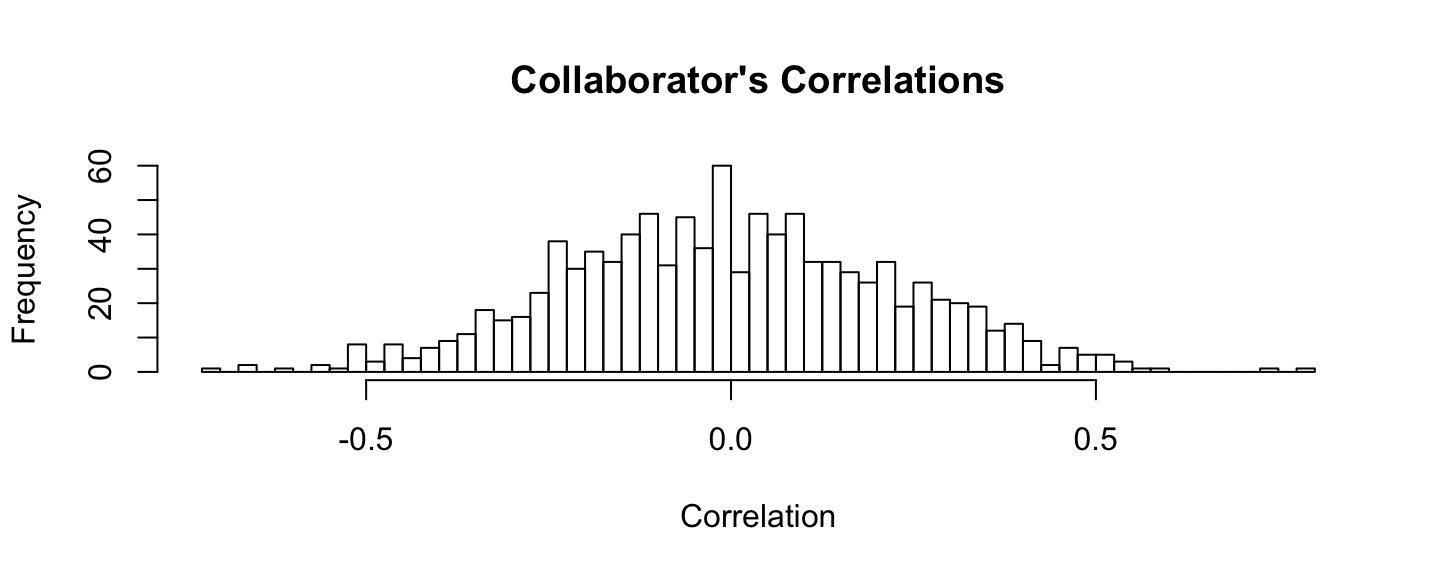## Example S9

Of course we don't expect to obtain the exact same correlations, but the pattern is fairly similar.

What about the regression on the top 10 genes?

## Example S10

Our ANOVA table:

```##           Df   Sum Sq Mean Sq  F value  Pr(>F)
## sigGenes  10 25.52398 2.55240 14.07385 0.00025
## Residuals  9  1.63222 0.18136```

Collaborator's ANOVA table:

```##           Df   Sum Sq Mean Sq  F value  Pr(>F)
## sigGenesC 10 16.91495 1.69150 13.82306 0.00027
## Residuals  9  1.10131 0.12237```

These are quite similar.

## Example S11

Similarly if we compute the 2-sample t-tests: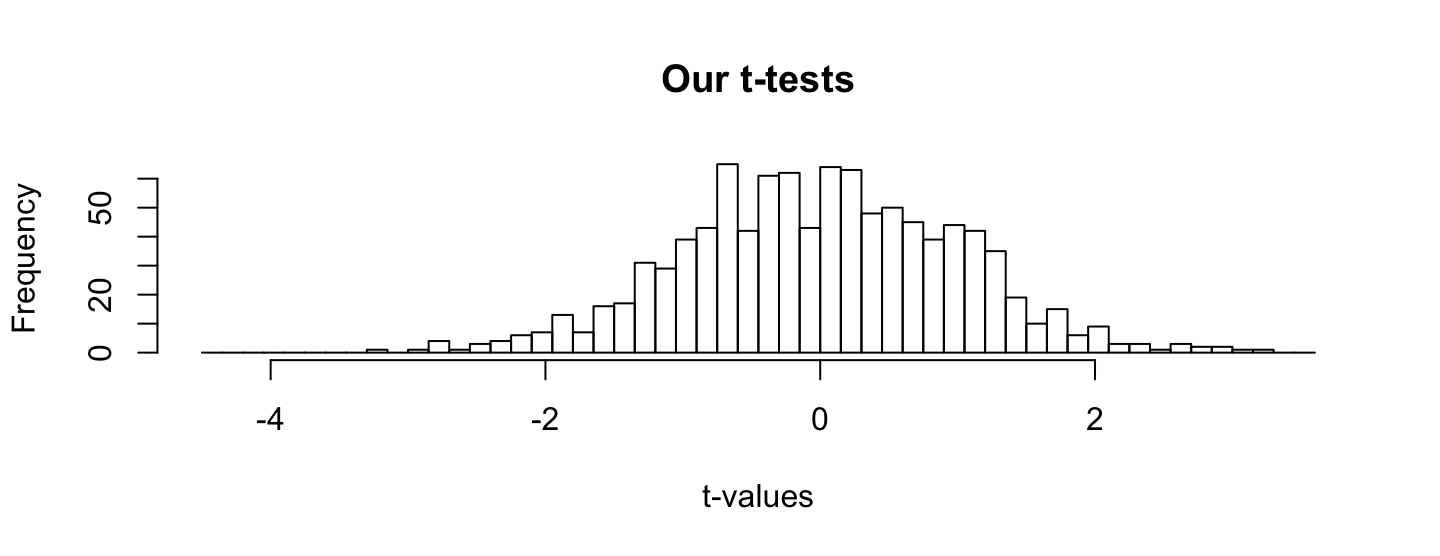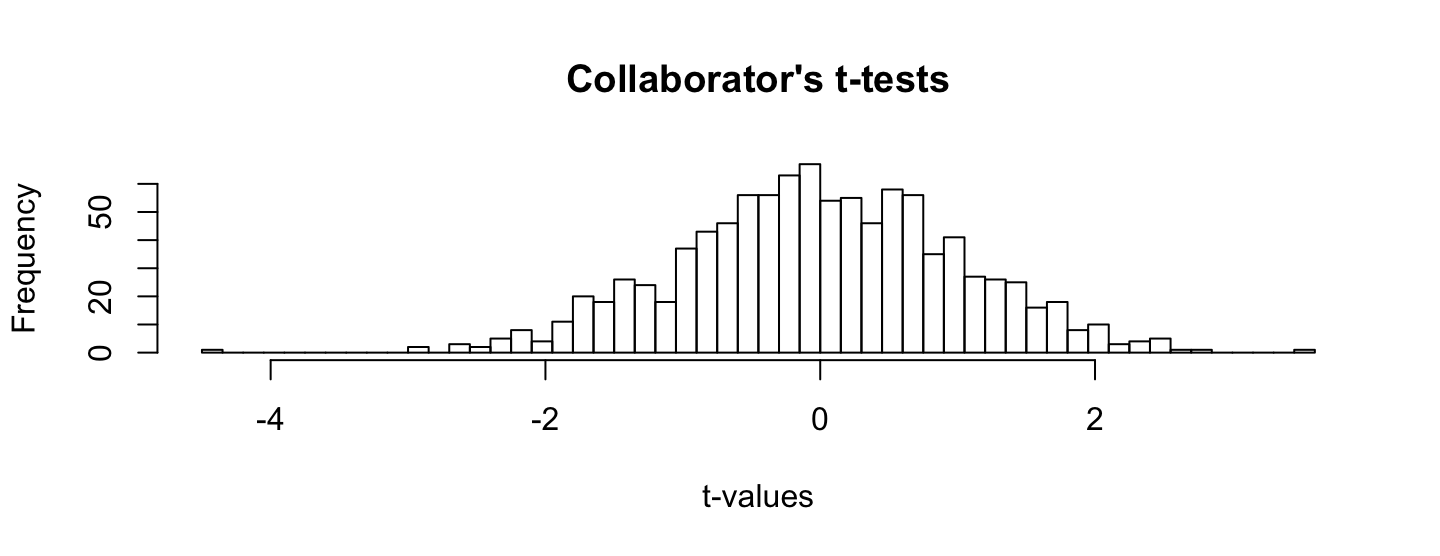## Example S12

The correspondance looks equally good if we consider a heatmap of gene expression and other typical measures.

But there is a problem -

Lets look e.g. at the genes with |cor|>.5 or p<0.05 for our study and our collaborator's study.

## Example S13

Here are the genes with correlation less than -0.5 or greater than 0.5.